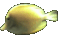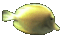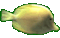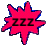Numbers & Variables in Expressions - Substitution Game Tips:

- In this game, replace the letter variable with the given number.

- For example, the expression (y+5) has the variable 'y' which can be replaced with a number.
If you are given y = 7 then you can substitute the number 7
into the expression (y+5) in order to get the answer (7+5) = 12.

- In this game, a variable such as 'y' represents a counting number.

- The word 'expression' in Mathematics has a very general meaning.
An expression can be any mathematical form using symbols.

- Your Game Score is reduced by the number of fish hits.

- To slow the game speed repeat tap/click on the word Slider.
- To increase the game speed repeat tap/click on the word Math.
- Speed can also be adjusted with a keyboard's - and + keys.

- Refresh/Reload the web page to restart the game.